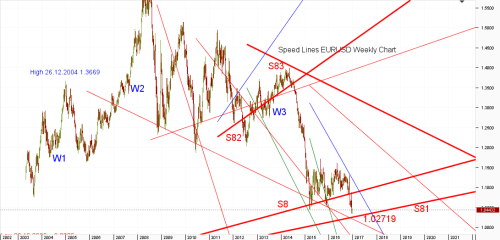## LOCInfo Custom Indicator follow the simple rules and make the right decision when to buy or sell. View Moving Average, Stochastic indicators from multiple time frames in one place.

• #1
• Topic Starter
Goal of this article is to explain the purpose, construction and implementation of speed lines, their use as trend lines for support and resistance and other possible uses derived from their implementation.

Purpose.
Properly constructed speed lines are important part of technical analysis, useful for both long term investors as well as traders because, they are well respected by price action of the security. As a result they often form strong support
or resistance and in many cases market reversal for considerable period of time.

Chart 1.Construction.
In this segment I will explain calculation of speed lines for long wave. For short waves the principle is the same, only reversed. Using octave system and half way principle, the question to solve was simple. Where would the price of the security be at this point of time or in the future, if it would proceed with 1/2, 1/4 or 1/8 of the original speed starting from the same point. The idea is the same as using 1/2, 1/4 or 1/8 of the price difference between high and low price of the wave, except instead of 3 horizontal price levels as a result we are getting 3 upper sloping lines. Including time in the equation is useful in many cases for retest levels where the line supports low values for second or third wave.

In this example we will calculate 1/8 speed line (S8) for wave W1 on weekly eurusd chart 1. Following data will be taken from daily chart.
Wave W1 Low 0.8229 Date 26.10.2000
High 1.3666 Date 30.12.2004

Difference 0.5437 \$ / 1526 days = 0.000356291 \$ / day.

Starting point of our speed line on 26.12.2004 is
Low + 1 / 8 of the price difference between high and low =
0.8229+(0.5437 / 😎 = 0.8908625

Reference point of our speed line on 26.11.2016 (can be any date after 30.12.2004) is
Low + (number of days from low x (\$/ day) / 😎 =
0.8229 + ( 5875 x 0.000356291 / 😎 =
0.8229 + 0.261651171= 1.08455.

Now we can implement on our chart trend line with following parameters.

Time 1 30.12.2004 Value 1 0.89086
Time 2 26.11.2016 Value 2 1.08455

Although 1/8 speed line S8 acted as a strong support, price of the instrument tends to reach 1/8 speed line from extreme levels, before reversing its course. In the case of eurusd it is line S81 which is 1/8 speed line of wave W2 which reached all time high after 94 months (important number, but that could be subject for another article).

Wave W2 low 0.8229 Date 26.10.2000
High 1.6038 Date 15.07.2008

Difference 0.7809 \$ / 2819 days = 0.000277013 \$ / day.

It is good practice to use current day as reference point, as it will give you price level for opening or closing position if the line is close to the price of the security. Using above equations and today date we can calculate parameters of the speed line.

Time 1 15.07.2008 Value 1 0.9205
Time 2 21.12.2016 Value 2 1.02719

Support and Resistance.
From the chart1 we can see that our speed line (S8)was effective support for 88 weeks.
As any support, once broken our speed line formed a resistance.
The line was tested as a resistance level first time on November 14th 2016, the same day the price gaped under it and second time on December 8th, 2016 24 days and 19 trading days later (= 1/2 of 38 another interesting number). To be fair it is notable to mention that our speed line was supported with mid line and Iv00 resistance line which can not be seen on the chart because they exactly line up with our speed line.

Chart 2.On chart 2 we can see speed lines on 15 minutes chart. To simplify the calculation, we can substitute bars for time. For example intra day calculation of 1/8 speed line R8 for short wave W3 on Chart 2 can be as follow.

W3 high 1.04795
low 1.03523 in 120 bars = 0.000106 \$ / bar

Start point of the line = high – ( high – low ) / 8 =
1.04795 – (1.04795 – 1.035223) / 8 = 1.04636

Reference point at 222th bar from high (red arrow point) =

high – (222 * pips/bar/ 😎 =
1.04795 – (222*0.000106 / 😎 = 1.0450085

Actual high reached was 1.04511.

Calculation for 1/4 and 1/2 speed lines are the same, except the divisions are by 2 and 4 respectively, instead of 8.

Possible uses.
If the speed line (S82) is part of corrective wave (W3), the wave often oscillates around it until it reaches previous wave speed line (S84) (Re Chart 1).
Crossovers of speed lines are principal time and price levels often marking important wave levels or midpoints (Re horizontal and vertical lines on Chart 2).
Mirror image of speed lines has the same effect on price of security as original speed lines.

Tips.
As you can see, the construction of speed lines can be time consuming,
and there fore if you are going to use them you will find useful to create script or another automated form of their construction.
For commodities, I would suggest implement speed lines based on cash price of the commodity as they work better even on commodity's future charts. (I have noticed significant difference especially in case of coffee).
In case of forex pairs and gold the difference is negligible. When constructing speed lines for longer time frames it is useful to check historical data of the security to avoid bar limitations of some platforms.